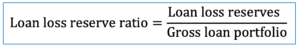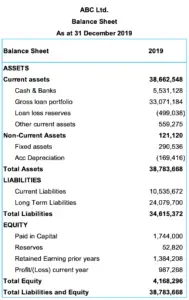# Loan Loss Reserve Ratio

## Overview

Loan loss reserve ratio is the ratio that is usually used in the bank or microfinance institution to indicate the reserve that the company makes in percentage to cover the estimated losses that it may suffer due to default loans.

In accounting, loan loss reserve is the contra account to gross loan outstanding. When the loan loss reserve is made, we make the journal entry by debit loan loss provision expense and credit loan loss reserves.

Loan loss reserve ratio can be used as an indication to measure the performance of the loan portfolio when compared with other companies in the same industry. However, as the reserve is an estimate, the comparison is usually only acceptable when the same accounting standard is applied.

## Loan Loss Reserve Ratio Formula

Loan loss reserve ratio can be calculated by using the formula of loan loss reserves dividing by gross loan portfolio.It is useful to note that loan loss reverse, loan loss allowance, and loan loss provision are the same thing in accounting. So, we may come across different wordings when we look at the financial statements of different companies.

However, the counterpart of loan loss reverses which we usually call it “loan loss provision expense” is different from loan loss reverses. Loan loss provision expense is an income statement item while loan loss reverses is a balance sheet item.

## Loan Loss Reserve Ratio Example

For example, we have the financial information of ABC Ltd. which is the microfinance institution in the form of balance sheet below:Calculate loan loss reserve ratio as at 31 December 2019

Solution:

With the financial information above, we can calculate loan loss reserve ratio as below:

Loan loss reserve = USD 499,038

Gross loan portfolio = USD 33,071,184

Loan loss reserve ratio = 499,038 / 33,071,184 = 1.51%

The excel calculation and data in the picture of the example above can be found in the link here: Loan loss reserve ratio calculation excel